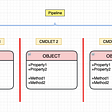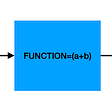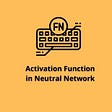# Greedy Algorithm

Greedy algorithms are an approach to solving certain kinds of optimization problems. Greedy algorithms are similar to dynamic programming algorithms in that the solutions are both efficient and optimal if the problem exhibits some particular sort of substructure. A greedy algorithm builds a solution by going one step at a time through the feasible solutions, applying a heuristic to determine the best choice. A heuristic applies an insight to solving the problem, such as always choose the largest, smallest, etc.

A greedy algorithm is an algorithm that follows the problem solving heuristic of making the locally optimal choice at each stage with the hope of finding a global optimum.

# Overview:

In general, greedy algorithms have five components:

1. A candidate (set, list, etc), from which a solution is created
2. A selection function, which chooses the best candidate to be added to the solution
3. A feasibility function, that is used to determine if a candidate can be used to contribute to a solution
4. An objective function, which assigns a value to a solution, or a partial solution, and
5. A solution function, which will indicate when we have discovered a complete solution

Greedy algorithms produce good solutions on some mathematical problems, but not on others.

Greedy algorithms should be applied to problems exhibiting these two properties:

• Greedy choice property We can make whatever choice seems best at the moment and then solve the subproblems that arise later. The choice made by a greedy algorithm may depend on choices made so far but not on future choices or all the solutions to the subproblem. It iteratively makes one greedy choice after another, reducing each given problem into a smaller one. In other words, a greedy algorithm never reconsiders its choices. This is the main difference from dynamic programming, which is exhaustive and is guaranteed to find the solution. After every stage, dynamic programming makes decisions based on all the decisions made in the previous stage, and may reconsider the previous stage’s algorithmic path to solution.
• Note:The traveling salesman problem doesn’t have this property, and therefore the greedy algorithm solution isn’t right for it.
• Optimal substructureA problem exhibits optimal substructure if an optimal solution to the problem contains optimal solutions to the sub-problems.

# Greedy Algorithm Making Change

Here we will determine the minimum number of coins to give while making change using a greedy algorithm. The coins in the U.S. currency uses the set of coin values {1,5,10,25}, and the U.S. uses a greedy algorithm which is optimal to give the least amount of coins as change. It is optimal because

• Total value of pennies: < 5 cents
• Total value of pennies and nickels: < 10 cents
• Total value of non-quarters: < 25 cents

Problems with greedy algorithm (when the greedy algorithm isn’t optimal):

1. Imagine a coin set of { 25-cent, 10-cent, 4-cent} coins. The greedy algorithm would not be able to make change for 41 cents, since after committing to use one 25-cent coin and one 10-cent coin it would be impossible to use 4-cent coins for the balance of 6 cents, whereas a person or a more sophisticated algorithm could make change for 41 cents with one 25-cent coin and four 4-cent coins.
2. Say we have {1,5,10,20,25} cents, what if we wanted minimum coins for 40 cents, the optimal choice will be two 20 cent coins, but the algorithm will choose coins 25,10,and 5, three coins.

Let’s use the greedy algorithm to give us the least amount of coins for 36 cents. We will use the coin set {1, 5, 10, 20}.

1. 36–20 = 16, List Solution: 20
2. 16–10 = 6, List Solution: 20, 10
3. 6–5 = 1, List Solution: 20, 10, 5
4. 1 -1 = 0, List Solution: 20, 10, 5, 1

# Code: Greedy Algorithm

Thanks for reading this article I hope its helpful to you all ! Keep up the learning, and if you would like more algorithm analysis videos please visit and subscribe to my YouTube channels (randerson112358 & compsci112358 )

# Computer Science Website:

http://everythingcomputerscience.com/

# Udemy Videos on Recurrence Relation:

Video Tutorial on Algorithm Analysis:
https://www.udemy.com/algorithm-analysis/

--

--

--

## More from randerson112358

Love podcasts or audiobooks? Learn on the go with our new app.

## CS373 Spring: Dillon Samra## Functional Shaders: A Colorful Intro-Part4 Gray Scale## Stop Accidentally Deleting Conversations in Messages on the Mac## Using In-Memory Databases in Windows — SQL Query Caching## Microsoft Teams “secret” Dev Tool## Technical Diagrams & Tools## PowerShell Pipeline## Traditional Programming VS Machine Learning Programming## Building a Convolutional Neural Network Using TensorFlow — Keras## Activation Function in Neural Network and Significance## Implementing Naive Bayes From Scratch in Python